# Pauli and Clifford Groups¶

## qecc.Pauli: Class representing Pauli group elements¶

The class qecc.Pauli is used to represent elements of the Pauli group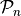on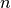qubits. Instances can be constructed by specifying strings of I, X, Y and Z, corresponding to the specification of an operator in the Pauli group.

>>> import qecc as q
>>> P = q.Pauli('X')
>>> print P
i^0 X
>>> Q = q.Pauli('XZZXI')
>>> print Q
i^0 XZZXI
>>> R = q.Pauli('XYZ')
>>> print R
i^0 XYZ


Additionaly, a phase can be provided. Since only integer powers ofare allowed as phases, the phase of a qecc.Pauli instance is represented by an integer in range(4). Any other integer is converted to an integer in that range that is equivalent mod 4.

>>> print q.Pauli('X', 2)
i^2 X


The qecc.Pauli class supports multiplication, tensor products and negation by the *, & and - operators, respectively.

>>> import qecc
>>> P = qecc.Pauli('X')
>>> Q = qecc.Pauli('Y')
>>> P * Q
i^1 Z
>>> P & Q
i^0 XY
>>> -P * Q
i^3 Z


Using these operators, it is straightforward to construct instances of qecc.Pauli from existing instances. To make this easier, QuaEC provides single-qubit operators I, X, Y and Z.

>>> from qecc import I, X, Y, Z
>>> print q.Pauli('XZZXI') & I
i^0 XZZXII


Additionally, instances of qecc.Pauli can be tested for equality.

>>> -P * Q == P * -Q
True
>>> P * Q != Q * P
True


The length of a qecc.Pauli is defined as the number of qubits it acts upon.

>>> print len(qecc.Pauli('XYZI'))
4


This information is also exposed as the property nq.

>>> print qecc.Pauli('XYZI').nq
4


### Class Reference¶

class qecc.Pauli(operator, phase=0)

Class representing an element of the Pauli group onqubits.

Parameters: operator (str) – String of I’s, X’s, Y’s and Z’s. phase (int) – A phase input as an integer from 0 to 3, interpreted as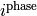.
nq

Returns the number of qubits upon which this Pauli operator acts.

wt

Measures the weight of a given Pauli.

Return type: int (between 0 and the number of qubits on which the Pauli is defined) The number of qubits on which the represented Pauli operator is supported.
str_sparse(incl_ph=True)

Returns a compact representation for qecc.Pauli objects, for those having support on a small number of qubits of a large register.

tens(other)

Concatenates the op strings of two Paulis, and multiplies their phases, to produce the Kronecker product of the two.

Parameters: other (qecc.Pauli) – Pauli operatorto be tensored with this instance. An instance representing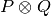, whereis the Pauli operator represented by this instance.
set_phase(ph=0)

Returns a qecc.Pauli object having the same operator as the input, with a specified phase (usually used to erase phases).

mul_phase(ph)

Increments the phase of this Pauli by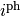.

Parameters: ph (int) – Amount the phase is to be incremented by. This instance.
permute_op(perm)

Returns a new qecc.Pauli instance whose operator part is related to the operator part of this Pauli, so that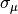is mapped tofor some permutation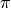of the objects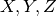.

For example:

>>> import qecc as q
>>> P = q.Pauli('XYZXYZ')
>>> print P.permute_op('ZXY')
i^0 ZXYZXY


Note that the result is not guaranteed to be the result of a Clifford operator acting on this Pauli, as permutation may not respect the phases introduced by taking products. For example:

>>> import qecc as q
>>> P = q.Pauli('XYZ')
>>> Q = q.Pauli('YYZ')
>>> P * Q
i^1 ZII
>>> Pp = P.permute_op('ZYX')
>>> Qp = Q.permute_op('ZYX')
>>> Pp * Qp
i^3 XII

Parameters: perm (list) – A list indicating which permutation is to be performed. A new instance Q of qecc.Pauli that is related to this instance by a permutation of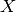,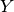and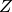.
as_gens()

Expresses an input Pauli in terms of the elementary generators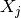and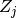, stripping off phases.

Return type: list of qecc.Pauli instances.
as_bsv()

Converts the given Pauli to a binary symplectic vector, discarding phase information.

Returns: A binary symplectic vector representing this Pauli operator. BinarySymplecticVector
as_circuit()

Transforms an n-qubit Pauli to a serial circuit on n qubits. Neglects global phases.

Return type: qecc.Circuit
as_unitary()

Returns a numpy.ndarray containing a unitary matrix representation of this Pauli operator.

Raises a RuntimeError if NumPy cannot be imported.

as_clifford()

Converts a Pauli into a Clifford which changes the signs of input Paulis. :returns: A Clifford representing conjugation by this Pauli operator. :rtype: qecc.Clifford

static from_sparse(sparse_pauli, nq=None)

Given a dictionary from non-negative integers to single-qubit Pauli operators or strings representing single-qubit Pauli operators, creates a new instance of qecc.Pauli representing the input.

>>> from qecc import Pauli, X, Y, Z
>>> print Pauli.from_sparse({3: X, 5: X, 7: Z}, nq=12)
i^0 X X Z

Parameters: sparse_pauli (dict) – Dictionary from qubit indices (non-negative integers) to single-qubit Pauli operators or to strings. nq (int) – If not None, specifies the number of qubits on which the newly created Pauli operator is to act.
static from_clifford(cliff_in)

Tests an input Clifford cliff_in to determine if it is, in fact, a Pauli. If so, it outputs the Pauli. If not, it raises an error.

Parameters: cliff_in – Representation of Clifford operator to be converted, if possible. qecc.Pauli

Example:

>>> import qecc as q
>>> cliff = q.Clifford([q.Pauli('XI',2),q.Pauli('IX')], map(q.Pauli,['ZI','IZ']))
>>> q.Pauli.from_clifford(cliff)
i^0 ZI


Converting a Pauli into a Clifford and back again will erase the phase:

>>> import qecc as q
>>> paul = q.Pauli('YZ',3)
>>> cliff = paul.as_clifford()
>>> q.Pauli.from_clifford(cliff)
i^0 YZ

static from_string(bitstring, p_1)

Creates a multi-qubit Pauli using a bitstring and a one-qubit Pauli, by replacing all instances of 1 in the bitstring with the appropriate Pauli, and replacing all instances of 0 with the identity.

Parameters: bitstring (list) – a list of integers, each of which is either 0 or 1. bitstring can also be a string, type conversion is automatic. p_1 (str) – a single-qubit Pauli. a phaseless Pauli from a bitstring. The intended use of this function is as a quick means of specifying binary Paulis. p_1 is the one_qubit Pauli that a ‘1’ represents. qecc.Pauli

Example:

>>> import qecc as q
>>> bitstring = '101110111100'
>>> p_1 = q.Pauli('X')
>>> q.Pauli.from_string(bitstring, p_1)
i^0 XIXXXIXXXXII

reg_wt(*args, **kwargs)

Produces the number of qubits within a subset of the register on which the Pauli in question acts non-trivially.

Parameters: region (tuple) – a tuple containing the indices on which the weight is to be evaluated. the number of qubits in the sub-register on which the Pauli self does not act as the identity.
cust_wt(char)

Produces the number of qubits on which an input Pauli acts as a specified single-qubit Pauli.

Parameters: char (str) – a single-letter string containing an I, X, Y or Z. the number of qubits in the Pauli self which are acted upon by the single-qubit operator char.
ct()

The conjugate transpose of this Pauli operator.

Return type: an instance of the qecc.Pauli class.
centralizer_gens(group_gens=None)

Returns the generators of the centralizer group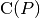, whereis the Pauli operator represented by this instance. If group_gens is specified,is taken to be a subgroup of the group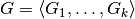, where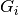is the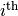element of group_gens.

Parameters: group_gens (list of qecc.Pauli instances) – Either None or a list of generators. If not None, the returned centralizeris a subgroup of the group. A list of elements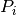of the Pauli group such that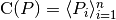, whereis the number of unique generators of the centralizer.
hamming_dist(other)

Returns the Hamming distance between this and another Pauli operator, defined as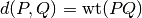.

### Iterating Over Groups and Subgroups¶

qecc.pauli_group(nq)

Generates an iterator onto the Pauli group ofqubits, whereis given as the argument nq.

Parameters: nq (int) – The number of qubits acted upon by the returned Pauli group. An iterator such that list(pauli_group(nq)) produces a list of all possible Pauli operators on nq qubits.
qecc.from_generators(gens, coset_rep=None, incl_identity=True)

Given a list of generators gens, yields an iterator onto the group generated by products of elements from gens.

If coset_rep is specified, returns the coset of the group generated by gens represented by coset_rep.

### Utility Functions¶

qecc.com(P, Q)

Given two elements P and Q of a Pauli group, returns 0 if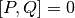and returns 1 if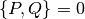.

Parameters: P (qecc.Pauli) – Representation of. Q (qecc.Pauli) – Representation of.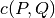. int
qecc.elem_gens(nq)

Produces all weight-oneandoperators on nq qubits. For example,

>>> import qecc as q
>>> Xgens, Zgens = q.elem_gens(2)
>>> print Xgens
i^0 IX

Parameters: nq (int) – Number of qubits for each returned operator. a tuple of two lists, containingandgenerators, respectively.
qecc.eye_p(nq)

Given a number of qubits, returns the identity Pauli on that many qubits.

Parameters: nq (int) – Number of qubits upon which the returned Pauli acts. qecc.Pauli A Pauli operator acting as the identity on each of nq qubits.

### Searching Over Pauli Group Elements¶

QuaEC provides useful tools for searching over elements of the Pauli group. A few particlar searches are provided built-in, while other searches can be efficiently built using the predicates described in Predicates and Filters.

qecc.is_in_normalizer(pauli, stab)

Given an element pauli of a Pauli group and the generators stab of a stabilizer group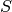, returns True if and only if pauli is in the normalizer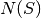.

qecc.mutually_commuting_sets(n_elems, n_bits=None, group_gens=None, exclude=None)

Yields an iterator onto tuples representing mutually commuting sets of n_elems independent Pauli operators, excluding the identity Pauli.

Parameters: n_elems (int) – The number of mutually commuting Pauli operators to include in each tuple. n_bits (int) – The number of qubits on which each Pauli operator considered by this iterator acts. If None, defaults to the number of qubits on which the first element of group_gens acts. group_gens (None or a sequence of qecc.Pauli instances) – The generators of the group in which to search for mutually commuting Pauli operators. Defaults to the elementary generators of the Pauli group on n_bits qubits. exclude (None or a sequence of qecc.Pauli instances) – If not None, the iterator will omit from its search any operators in the group generated by exclude.

## qecc.Clifford: Class representing Clifford group elements¶

Elements of the automorphism group of the Pauli group (known as the Clifford group) are represented by the class qecc.Clifford. Instances of Clifford are constructed by specifying the mappings of the generators of the Pauli group, such that the action of a Clifford instance is defined for all input Pauli group elements.

>>> import qecc as q
>>> C = q.Clifford(['XX', 'IX'], ['ZI', 'ZZ'])
>>> print C
XI |->  +XX
IX |->  +IX
ZI |->  +ZI
IZ |->  +ZZ


Also, the results of an element of the Clifford group can be left partially unspecified, using the singleton qecc.Unspecified:

>>> import qecc as q
>>> print q.Clifford(['IZ','XZ'],['XI',q.Unspecified])
XI |->  +IZ
IX |->  +XZ
ZI |->  +XI
IZ |-> Unspecified


Once an instance of qecc.Clifford has been constructed in this way, its action on elements of the Pauli group can be calculated by calling the Clifford instance as a function.

>>> from qecc import I, X, Y, Z
>>> C(X & Y)
i^0 YZ
>>> map(C, ['XI', 'IX', 'YI', 'IY', 'ZI', 'IZ'])
[i^0 XX, i^0 IX, i^0 YX, i^0 ZY, i^0 ZI, i^0 ZZ]


Note that in this example, C has converted strings to qecc.Pauli instances. This is done automatically by qecc.Clifford.

Instances of Clifford can be combined by multiplication (*) and by tensor products (&). Multiplication of two Clifford instances returns a new instance representing their composition, while the tensor product returns a new instance that acts on each register independently.

>>> import qecc as q
>>> C = q.Clifford(['XX', 'IX'], ['ZI', 'ZZ'])
>>> D = q.Clifford(['XI', 'IZ'], ['ZI', 'IX'])
>>> print C * D
XI |->  +XX
IX |->  +ZZ
ZI |->  +ZI
IZ |->  +IX
>>> print C & D
X |->  +X X
X |->  +Z
Z |->  +Z Z
Z |->  +X


Note that in the second example, the printing of the Clifford operator has switched to a sparse format that suppresses printing lines for qubits that are not acted upon by the operator (in this case, qubits 1 and 2 are trivially acted upon by C & D).

As with qecc.Pauli, the length of a qecc.Clifford instance is defined as the number of qubits on which that instance acts. This information is also exposed as the property nq.

>>> import qecc as q
>>> C = q.Clifford(['XX', 'IX'], ['ZI', 'ZZ'])
>>> print len(C)
2
>>> print C.nq
2


### Class Reference¶

class qecc.Clifford(xbars, zbars)

Class representing an element of the Cifford group onqubits.

Parameters: xbars (list of qecc.Pauli instances) – A list of operators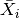such that the represented Clifford operation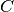acts as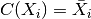. Note that in order for the represented operator to be an automorphism, eachmust have phase either 0 or 2. A warning will result if this condition is not met. zbars (list of qecc.Pauli instances) – See xbars.
nq

Returns the number of qubits on which a qecc.Clifford object acts.

n_unspecified

Returns the number of unspecifed outputs of a qec.Clifford object.

str_sparse()

Provides a compact representation for qecc.Clifford objects in the case where many of the outputs have small support.

is_valid(quiet=True)

Returns True if this instance represents a valid automorphism. In particular, this method returns True if all output phase assignments are either 0 or 2, and if all of the commutation relations on its outputs are obeyed. Unspecified outputs are ignored.

Parameters: quiet (bool) – If set to True, this method will not print out any information, but will return True or False as described above. Otherwise, if the operator is not a valid Clifford operator, diagnostic information will be printed.
inv()

Calculates the inverse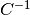of this Clifford operator, such thatis the identity Clifford.

conjugate_pauli(pauli)

Given an instance of qecc.Pauli representing the operator, calculates the mapping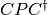, whereis the operator represented by this instance.

Parameters: pauli (qecc.Pauli) – Representation of the Pauli operator. Representation of the Pauli operator, whereis the Clifford operator represented by this instance. qecc.Pauli
constraint_completions()

Yields an iterator onto possible Clifford operators whose outputs agree with this operator for all outputs that are specified. Note that all yielded operators assign the phase 0 to all outputs, by convention.

If this operator is fully specified, the iterator will yield exactly one element, which will be equal to this operator.

For example:

>>> import qecc as q
>>> C = q.Clifford([q.Pauli('XI'), q.Pauli('IX')], [q.Unspecified, q.Unspecified])
>>> it = C.constraint_completions()
>>> print it.next()
XI |->  +XI
IX |->  +IX
ZI |->  +ZI
IZ |->  +IZ
>>> print it.next()
XI |->  +XI
IX |->  +IX
ZI |->  +ZI
IZ |->  +IY
>>> print len(list(C.constraint_completions()))
8


If this operator is not a valid Clifford operator, then this method will raise an qecc.InvalidCliffordError upon iteraton.

as_bsm()

Returns a representation of the Clifford operator as a binary symplectic matrix.

Return type: qecc.BinarySymplecticMatrix
as_unitary()

Returns a numpy.ndarray containing a unitary matrix representation of this Clifford operator.

Raises a RuntimeError if NumPy cannot be imported.

circuit_decomposition()

Returns a qecc>Circuit object consisting of the circuit decomposition of self.as_bsm() and a qecc.Pauli object which ensures the output phases of the qecc.Clifford object are preserved.

### Alternate Constructors¶

In addition to specifying the outputs of a Clifford operator acting on the elementary generators of the Pauli group, one can also create a Clifford instance by specifying the ouput of an operator on an arbitrary generating set. In particlar, the function qecc.generic_clifford() takes the inputs and outputs of a given Clifford operator in order to create a qecc.Clifford instance.

qecc.generic_clifford(paulis_in, paulis_out)

Given two lists of qecc.Pauli instances, paulis_in and paulis_out, produces an instance C of qecc.Clifford such that C(paulis_in[i]) == paulis_out[i] for all i in range(2 * nq), where nq is the length of each element of the two lists.

Each of paulis_in and paulis_out is assumed to be ordered such that the slice [0:nq] produces a list of logicaloperators, and such that the slice [nq:2*nq] produces the logicaloperators.

Parameters: paulis_in – A list of length 2 * nq logical Pauli operators specifying the input constraints for the desired Clifford operation. paulis_out – A list of length 2 * nq logical Pauli operators specifying the output constraints for the desired Clifford operation. A Clifford operator mapping the input constraints to the output constraints. qecc.Clifford

### Iterators onto the Clifford Group¶

qecc.clifford_group(nq, consider_phases=False)

Given a number of qubits, returns an iterator that produces all elements of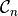, the Clifford group onqubits.

Parameters: nq (int) – The number of qubits upon which each yielded element will act. consider_phases (bool) – If True, then Clifford operators whose assignments of phases to the generators of the Pauli group differ will be treated as distinct. Otherwise, the yielded elements will be drawn from the group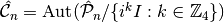, such that the phases of the outputs are not considered.

### Common Clifford Gates¶

The qecc package provides support for several common Clifford operators. These functions can be used to quickly analyze small circuits. For more extensive circuit support, please see Circuit Manipulation and Simulation.

qecc.eye_c(nq)

Yields the identity Clifford, defined to map every generator of the Pauli group to itself.

Return type: Clifford
qecc.cnot(nq, ctrl, targ)

Yields the nq-qubit CNOT Clifford controlled on ctrl, acting a Paulion targ.

Return type: qecc.Clifford

Yields the nq-qubit Clifford, switchingandon qubit q, yielding a minus sign on.

Return type: qecc.Clifford
qecc.phase(nq, q)

Yields the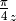-rotation Clifford, acting on qubit q.

Return type: qecc.Clifford
qecc.swap(nq, q1, q2)

Yields the swap Clifford, on nq qubits, which swaps the Pauli generators on q1 and q2.

Return type: qecc.Clifford
qecc.cz(nq, q1, q2)

Yields the nq-qubit C-Z Clifford, acting on qubits q1 and q2.

Return type: qecc.Clifford
qecc.pauli_gate(pauli)

Imports an instance of the qecc.Pauli class into the qecc.Clifford class, representing a Pauli as a series of sign changes.

Return type: qecc.Clifford